Back to Articles

# The How and Why of Fast Fourier Transform (FFT) Analysis

#### Analysis

Vibration occurs in the time domain, meaning it oscillates over time. After collecting vibration data, it is often advantageous to transform it into a different domain for analysis. Patterns not apparent in the time domain are visible in others, giving engineers a clearer understanding of their product’s vibrational behavior.

### Fast Fourier Transform

Engineers often analyze vibration as a function of frequency. The fast Fourier transform (FFT) transforms time-domain data into the frequency domain by deconstructing the signal into sine and cosine waves. It is a computational algorithm that efficiently implements a mathematical operation called the discrete-time Fourier transform (DTFT).

The FFT helps engineers determine the excitation frequencies in a complex signal and their amplitude. It also highlights changes in frequency and amplitude and harmonic excitation in the selected frequency range.

For a complex signal, the FFT can help to answer:

• What frequencies are being excited?
• What is the amplitude at each frequency?
• What changes throughout the waveform?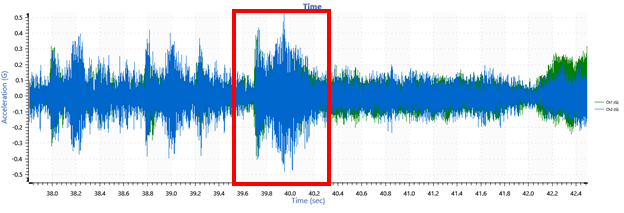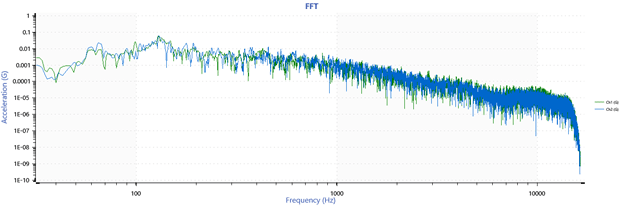A segment of time-domain data projected onto the frequency domain.

### Simplified Explanation of Fourier Analysis

In general terms, the foundation of the frequency domain is made up of sine and cosine waveforms. The Fourier series works on the principle that we can construct any signal by the sum of a series of sine and cosine waves.

Algorithms can analyze (or separate) time data into components by projecting it onto these sine and cosine waveforms. The set of these components for the given signal is a transform.

The Fourier transform takes apart data using projections. In practice, it digitizes the signal, x, into a sequence of N numbers (xn, n=1 to N) with a time interval of Δt between samples. The resulting set of components is the Fourier transform of x(t).

For a more comprehensive explanation, visit the following VRU lessons: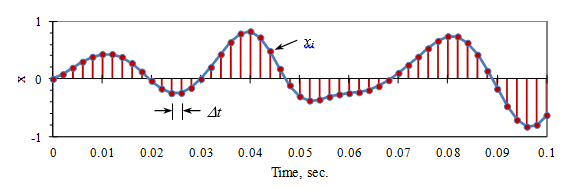Digitization of a time signal.

#### The FFT Speeds Things Up

The DTFT projects a time data sequence of N length onto sinusoids at N frequencies. The FFT performs the same mathematical operation as the DTFT with computational efficiency. Its algorithm uses a sequence of N digital samples where N is a power of 2 (2, 4, 8, 16, 32, etc.).

For example, if a data sequence has a length of N=1,024, a DTFT operation would require about N2=1,048,576 operations, whereas the FFT would require about Nlog2N=10,240 operations (about one-tenth as many).

However, this efficiency creates a constraint—the FFT requires the length N to be a power of 2. As such, all analysis line values must also be a power of 2.

### FFT Analysis in ObserVIEW

FFT analysis in the ObserVIEW software is an efficient method of data analysis. The software responds to any change to the settings with a fast and automatic recalculation of the computed parameters. The software can perform the calculation on live data streaming from Live Analyzer or an imported file.

Features of ObserVIEW’s FFT analysis include:

• Up to 1,048,576 analysis lines (user-defined)
• Industry-standard window functions with comprehensive support for the selection process
• Standard, harmonic, and RMS cursor types and more
• Quick reporting features, including copy-paste functionality
• Live computation of data with Live Analyzer
• AVD conversion (acceleration, velocity, displacement)

### FFT Parameters

To add an FFT graph in ObserVIEW, select Add Graph > FFT. The user-defined settings include analysis lines, window function, and data bias range.

#### Analysis Lines

The sample rate and analysis lines determine the frame length of an FFT. Fewer lines will result in a shorter frame of time data, and more lines will result in a longer frame of time data.

The length of each frame required to generate an FFT is (analysis lines *2)/sample rate. For pure FFT analysis, the frequency resolution is (sample rate/2)/analysis lines. If the FFT resolution is known, the test engineer can also use 1/FFT resolution to equate the time duration of each FFT frame.

Increasing the number of analysis lines increases the FFT frequency resolution, which is useful when analyzing low-frequency content. Increasing this parameter also results in a higher resolution of the resulting analysis plot. However, higher lines increase the computational burden, and the increased analysis time can result in a slower response to change.

#### Window Function

The FFT calculation interprets the two endpoints of a time waveform as though they were connected—i.e., they have the same value at each end of a time interval. However, random signals are rarely equal at each end of the time interval. This assumption creates discontinuities in the time domain and results in unwanted noise in the frequency spectrum.

Window functions are added to the signal-processing algorithm to address these discontinuities. ObserVIEW includes the industry-standard window functions including Blackman, Hamming, and Hanning. The user should select the function that best fits the application or the purpose of the analysis. Here are a few tips when selecting a window function:

• Select Blackman, Hamming, or Hanning for general vibration analysis
• Select Rectangular for periodic signals that are shorter than the window length
• Flat Top provides very accurate amplitude measurements, but the user sacrifices frequency accuracy for better amplitude accuracy
• Select Exponential for impact testing, burst random shock, and shock analysis

The ObserVIEW Help file also includes a comprehensive table of window functions for assistance with selection.

#### Cursors and Annotations

ObserVIEW includes a multitude of single-value and differential cursors for analysis. The standard cursor displays the trace values at the cursor location. Cursors such as harmonic and RMS can be of value during FFT analysis to determine the trace values at each harmonic of the base cursor and calculate the RMS trace values between the base and primary cursors.

#### Copy Paste Feature

The copy and paste command in ObserVIEW allows the user to move data between graphs and software applications. In doing so, the user can perform an easy comparison of data from a multitude of applications. This can be useful for comparing graph data from different time locations, multiple test results, various test environments, and much more.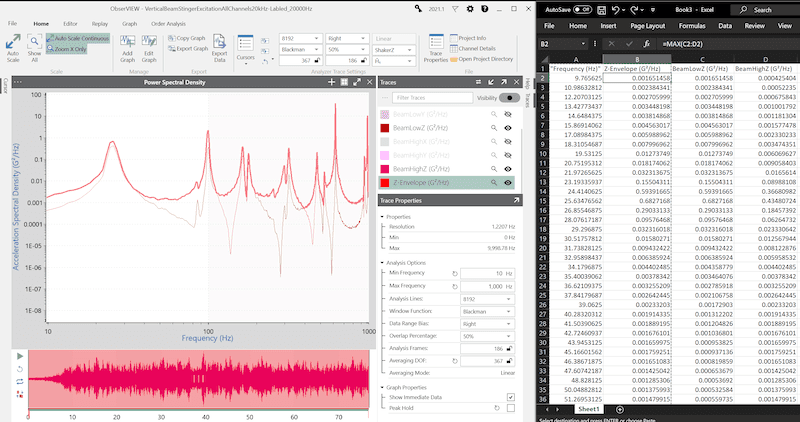Within the ObserVIEW software, graph traces can be copied and pasted to any graph to create a static trace, including the same graph from which it was copied. This option can be beneficial when comparing FFTs from different time locations.

If the user would like to compare data from a graph in VibrationVIEW to data in ObserVIEW, they can do so with the copy and paste command. A graph or trace from ObserVIEW can also be copied and pasted into Microsoft Excel or another text document application. In Excel, the graph data is pasted, and in Word, the image of the graph is pasted.

The user can also copy/paste data from other sources (Excel, TXT, CSV, etc.) into ObserVIEW to create visual reference lines on any graph.

### Live Analyzer

When a device is connected to a Live Analyzer session, the user can access all analysis controls while live data are streamed in from the device. The user can pause the incoming data to perform a more in-depth analysis and/or export the waveform. They can then resume the live feed without losing any of the data that occurred while the stream was paused so long as the data was paused for less than the user-configurable buffer duration period.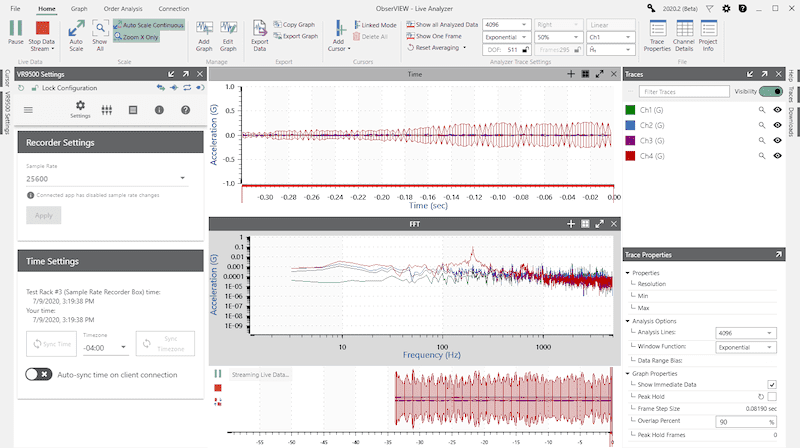Download the free demo of ObserVIEW today and begin analyzing and editing waveforms straight away. Interested in learning more? Visit the software page.

Date

May 12, 2023

Author

Cherie Stoll

Category